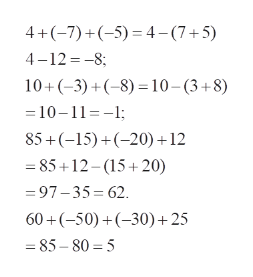4 + (-7) + (-5)=10 + (-3) + (-8)=85 + (-15) + (-20) + 12=60 + (-50) + (-30) + 25=17 + (-4) + 2 + 3 + (-10)= 19 + (-5) + 1 + 8 + (-13)=-8a + (-15a)=-9a + (-13a)=

Question

4 + (-7) + (-5)=

10 + (-3) + (-8)=

85 + (-15) + (-20) + 12=

60 + (-50) + (-30) + 25=

17 + (-4) + 2 + 3 + (-10)=

19 + (-5) + 1 + 8 + (-13)=

-8a + (-15a)=

-9a + (-13a)=

Step 1

To simplify the given additions and subtractions:

Step 2

As a general principle, group the positive terms and negative terms separately  and simplify

Step 3help_outlineImage Transcriptionclose4+(-7)(5)4-(7+5) 4 12 -8 10+(3)(8) 10-(3+8) =10-11--1; 85+(15)(20 +12 85 12-15 20) =97-35 62 60 (50)(30)+25 = 85-80 5 fullscreen

Want to see the full answer?

See Solution

Want to see this answer and more?

Our solutions are written by experts, many with advanced degrees, and available 24/7

See Solution
Tagged in

Algebra